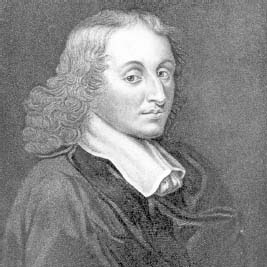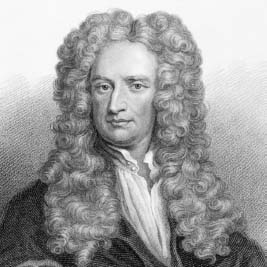NextPrevious

# Who began the mathematical study of probability?

French scientist and religious philosopher Blaise Pascal (1623–1662) is known not only for the study of probability, but many other mathematical-oriented advances, such as a calculation machine (invented at age 19 to help his father with tax calculations, but it performed only additions), hydrostatics, and conic sections. He is also credited (along with Fermat) as the founder of modern theory of probability. (For more information about probability, see “Applied Mathematics.”)Seventeenth-century scientist Blaise Pascal was the founder of mathematical probability, as well as other achievements, such as devising one of the first calculating machines.Most people know about Sir Isaac Newton’s famous laws of motion. He also invented differential and integral calculus.

Close

This is a web preview of the "The Handy Math Answer Book" app. Many features only work on your mobile device. If you like what you see, we hope you will consider buying. Get the App